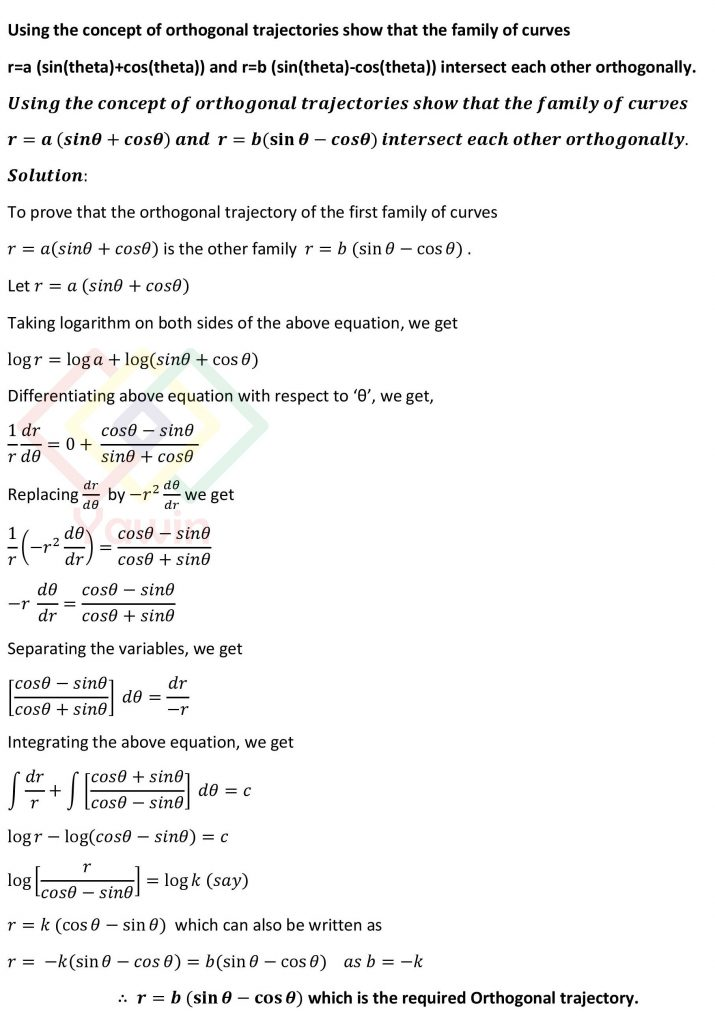Using the concept of orthogonal trajectories show that the family of curves r=a (sin(theta)+cos(theta)) and r=b (sin(theta)-cos(theta)) intersect each other orthogonally

## Question## Question

Using the concept of orthogonal trajectories show that the family of curves r=a (sin(theta)+cos(theta)) and r=b (sin(theta)-cos(theta)) intersect each other orthogonally

## Topic

Applications of Differential Equations